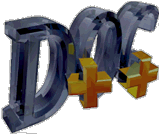## Classes

• AbstractTable Interface for associative array, or hash table, data access
• HCL_Adjoint_d HCL_Adjoint_d creates the adjoint operator of an HCL_LinearOp_d as an operator in its own right
• HCL_ALFcnl_d HCL_ALFcnl_d implements an Augmented Lagrangian functional; it is intended for use with HCL_CMinAL_d
• HCL_Base HCL_Base is the base class for all HCL classes
• HCL_BigMFcnl_d HCL_BigMFcnl_d implements a "big-M" functional for the problem of finding a vector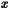satisfying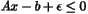(that is, the goal is to find a pointstrictly satisfying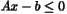)
• HCL_BigMFcnlHessian_d HCL_BigMFcnlHessian_d implements the Hessian of the big-M functional represented by HCL_BigMFcnl_d as an object derived from HCL_LinearOp_d
• HCL_BiLinearOp_d HCL_BiLinearOp_d is the base class for bilinear operators
• HCL_BlockBiLinearOp_d HCL_BlockBiLinearOp_d implements a bilinear operator of the following form: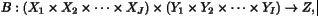, where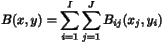and each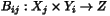is a bilinear operator
• HCL_BlockLinearOp_d HCL_BlockLinearOp_d implements a 2D array of linear operators acting on a product vector space
• HCL_CG_d HCL_CG_d implements the (unpreconditioned) conjugate gradients algorithm for solving a linear operator equation with a symmetric positive definite operator
• HCL_CMinAL_d HCL_CMinAL_d implements the augmented Lagrangian algorithm for an equality constrained nonlinear programs
• HCL_CompLinearOp_d HCL_CompLinearOp_d is a concrete class implementing a composition of two or more linear operators
• HCL_DiagBlockLinearOp_d HCL_DiagBlockLinearOp_d implements a diagonal array of linear operators (with adjoints and inverses) acting on a product vector space
• HCL_DiagScaleLinearOp_d HCL_DiagScaleLinearOp_d is a class implementing the linear operator mapping x to a*x, where x and a are vectors and the multiplication is done componentwise (in "Matlab notation", this operation is a
• HCL_EvalALFcnl_d HCL_EvalALFcnl_d is the evaluation object for the class HCL_ALFcnl_d, which implements an Augmented Lagrangian functional
• HCL_EvalBigMFcnl_d HCL_EvalBigMFcnl_d is the "evaluation object" for the functional class HCL_BigMFcnl_d, which implements a "big-M" functional for the problem of finding a vectorsatisfying(that is, the goal is to find a pointstrictly satisfying)
• HCL_EvalFunctionalProductDomain_d HCL_EvalFunctionalProductDomain_d is the evaluation object for the HCL_FunctionalProductDomain_d class
• HCL_EvalLinCombFcnl_d This class represents the "evaluation" object for HCL_LinCombFcnl_d, which implements a linear combination of real-valued functionals: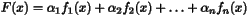• HCL_EvalLNLOp_d HCL_EvalLNLOp_d is the evaluation object for HCL_LNLOp_d
• HCL_EvalLSFcnl_d HCL_EvalLSFcnl_d is the evaluation object for the functional class HCL_LeastSquareFcnl_d, which implements a least-squares functional based on a nonlinear operator
• HCL_EvalLSFcnlGN_d HCL_EvalLSFcnlGN_d is the evaluation object for the functional class HCL_LeastSquareFcnlGN_d, which implements a least-squares functional based on a nonlinear operator
• HCL_EvalLSFcnlGNProductDomain_d HCL_EvalLSFcnlGNProductDomain_d is the evaluation object for the functional class HCL_LeastSquareFcnlGNProductDomain_d, which implements a least-squares functional based on a nonlinear operator
• HCL_EvalLSFcnlProductDomain_d HCL_EvalLSFcnlProductDomain_d is the evaluation object for the functional class HCL_LeastSquareFcnlProductDomain_d, which implements a least-squares functional based on a nonlinear operator
• HCL_EvalOpProductDomain_d This class represents an "evaluation" object that will be created by an HCL_OpProductDomain_d object to implement the image and derivatives of the operator at a point
• HCL_EvalQuadFunctional_d HCL_EvalQuadFunctional_d is the evaluation object for HCL_QuadFunctional_d, which implements a functional of the form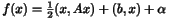• HCL_EvalRestrictedFunctional_d HCL_EvalRestrictedFunctional_d is the evaluation class for HCL_RestrictedFunctional_d, which is the concrete class which turns a functional defined on a product space into an ordinary functional by fixing all but one of the components
• HCL_EvaluateFunctional_d This class represents an "evaluation" object that will be created by a instance of HCL_Functional object to implement the value, gradient, and Hessian of the functional at a point
• HCL_EvaluateOp_d This class represents an "evaluation" object that will be created by an HCL_Op_d object to represent the image and derivatives of the operator at a point
• HCL_FPArch_d HCL_FPArch_d is an interface class to the LAPACK DLAMCH, which determines the floating point characteristics of machine on which the code is running
• HCL_Functional_d HCL_Functional is the base class for all real-valued functions
• HCL_FunctionalDefaultEval_d The purpose of the class HCL_FunctionalDefaultEval is to relieve the implementor of a derived functional class from the necessity of also defining a derived evaluation class if there is no need to do so
• HCL_FunctionalDefaultHessian_d The purpose of the class HCL_FunctionalDefaultHessian is to relieve the implementor of a derived functional class from the necessity of also defining a class to represent the Hessian if there is no existing HCL class that can be used for this purpose
• HCL_FunctionalDefaultHessianBlock_d The purpose of the class HCL_FunctionalDefaultHessianBlock is to relieve the implementor of a derived functional class from the necessity of also defining a class to represent blocks of the Hessian if there is no existing HCL class that can be used for this purpose
• HCL_FunctionalProductDomain_d HCL_FunctionalProductDomain_d is an abstract base class used to represents functionals defined on product spaces
• HCL_FunctionalProductDomainDefaultEval_d The purpose of the class HCL_FunctionalProductDomainDefaultEval is to relieve the implementor of a derived functional class from the necessity of also defining a derived evaluation class if there is no need to do so
• HCL_GeneralMatOp_d HCL_GeneralMatOp_d makes a matrix into a linear operator in the HCL sense
• HCL_GenericProductSpace_d HCL_GenericProductSpace_d is a concrete product vector space class; it represents a vector space which is defined as a product of several existing vector spaces
• HCL_GenericProductVector_d HCL_GenericProductVector_d is a concrete product vector class; it represents a vector from a vector space which is defined as a product of several existing vector spaces
• HCL_InvLinearOp_d HCL_InvLinearOp_d creates the inverse operator of a HCL_LinearOp_d as an operator in its own right
• HCL_IRArnoldi_d HCL_IRArnoldi_d implements the implicitly restarted Arnoldi algorithm for solving the (generalized) eigenvalue problem
• HCL_ItEigSolver_d Abstract base class for iterative eigenvalue solvers
• HCL_LeastSquaresFcnl_d HCL_LeastSquaresFcnl_d creates least squares objective functions from operators and data
• HCL_LeastSquaresFcnlGN_d HCL_LeastSquaresFcnlGN creates least squares objective functions from operators and data
• HCL_LeastSquaresFcnlGNProductDomain_d HCL_LeastSquaresFcnl_ creates least squares objective functions from operators and data
• HCL_LeastSquaresFcnlProductDomain_d HCL_LeastSquaresFcnlProductDomain_d creates least-squares objective functions from operators and data
• HCL_LinCombFcnl_d HCL_LinCombFcnl_d represents a linear combination of real-valued functionals:• HCL_LinCombLinearOp_d HCL_LinCombLinearOp_d is a concrete class implementing a linear combination of one or more linear operators
• HCL_LinearOp_d HCL_LinearOp_d is the base class for all linear operators
• HCL_LinearSolver_d HCL_LinearSolver_d is the base class for linear solvers, that is, for objects representing algorithms for solving linear operator equations
• HCL_LineSearch_d Abstract base class for line search routines
• HCL_LineSearch_DS_d An implementation of a backtracking line search algorithm using cubic interpolation (See Dennis and Schnabel, "Numerical Methods for Unconstrained Optimization and Nonlinear Equations", Prentice-Hall (1983))
• HCL_LineSearch_Fl_d An implementation of a backtracking line search algorithm using cubic and quadratic interpolation (See Fletcher, "Practical Methods of Optimization" (2nd edition), Wiley, (1987))
• HCL_LineSearch_MT_d An implementation of the More' and Thuente line search algorithm (See More' and Thuente, "Line Search Algorithms with Guaranteed Sufficient Decrease", ACM TOMS, Vol
• HCL_lmbfgsOp1_d HCL_lmbfgsOp1_d implements the limited memory BFGS approximation to the inverse Hessian of a twice-differentiable function
• HCL_LNLOp_d HCL_LNLOp_d creates a nonlinear op from a linear one
• HCL_LowerTriangularMatOp_d HCL_LowerTriangularMatOp_d makes an lower triangular matrix into a linear operator in the HCL sense
• HCL_MatTR_d HCL_MatTR_d implements an algorithm for solving the trust region subproblem in the case in which the operator is represented by a symmetric matrix
• HCL_Normal_d HCL_Normal_d creates the normal operator of a HCL_LinearOp_d as an operator in its own right
• HCL_Op_d HCL_Op_d is the base class for (presumably nonlinear) operators
• HCL_OpDefaultDeriv_d HCL_OpDefaultDeriv_d is a linear operator class implementing the derivative of a nonlinear operator
• HCL_OpDefaultEval_d HCL_OpDefaultEval_d is the default "evaluation" class for nonlinear operators
• HCL_OpDefaultPartialDeriv_d HCL_OpDefaultPartialDeriv_d is a linear operator class implementing a partial derivative of a nonlinear operator
• HCL_OpDefaultSecondDeriv_d HCL_OpDefaultSecondDeriv_d is a bilinear operator class implementing the second derivative of a nonlinear operator
• HCL_OpDefaultSecondPartialDeriv_d HCL_OpDefaultSecondPartialDeriv_d is a linear operator class implementing a second partial derivative of a nonlinear operator
• HCL_OpPDDefaultEval_d HCL_OpPDDefaultEval_d is the default "evaluation" class for nonlinear operators defined on product spaces
• HCL_OpProductDomain_d HCL_OpProductDomain_d is the base class for (presumably nonlinear) operators
• HCL_PCG_d HCL_PCG_d implements the preconditioned conjugate gradients algorithm for solving a linear operator equation with a symmetric positive definite operator
• HCL_ProductSpace_d HCL_ProductSpace_d is the base class for product vector space classes
• HCL_ProductVector_d HCL_ProductVector_d represents a vector from a product space, that is, from a space of the form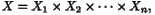where each of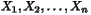is a vector space represented by an instance of HCL_VectorSpace_d
• HCL_QuadFunctional_d HCL_QuadFunctional_d implements a quadratic functional defined as follows: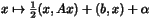• HCL_Recorder_d HCL_Recorder defines an interface for "recorder" objects, used principally for recording intermediate results during an iterative process
• HCL_RestrictedBiLinearOp_d HCL_RestrictedBiLinearOp_d creates one of the three linear operators related to a bilinear operator
• HCL_RestrictedFunctional_d HCL_RestrictedFunctional_d is a concrete class which creates a new functional from a functional defined on a product space, by fixing all but one of the components of the independent variable
• HCL_RnSpace_d HCL_RnSpace_d is a vector space class representing the simplest vector space, namely, Euclidean n-space
• HCL_RnVector_d HCL_RnVector_d is the simplest vector class
• HCL_ScaleLinearOp_d HCL_ScaleLinearOp_d implementing the linear operator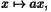where a is a scalar
• HCL_SparseRCSMatOp_d HCL_SparseRCSMatOp_d makes a sparse matrix into a linear operator in the HCL sense
• HCL_SymMatOp_d HCL_SymMatOp_d makes a symmetric matrix into a linear operator in the HCL sense
• HCL_SymSparseRCSMatOp_d HCL_SymSparseRCSMatOp_d makes a sparse symmetric matrix into a linear operator in the HCL sense
• HCL_TRCG_d The class HCL_TRCG_d is an implentation of the Steihaug-Toint algorithm for approximately solving the trust region subproblem
• HCL_TRSSOp_d The class HCL_TRSSOp_d defines the bordered operator used by HCL_TrustRegion_SS_d
• HCL_TrustRegion_SS_d NOTE: This class is experimental and has not been carefully debugged
• HCL_TrustRegionSolver_d The class HCL_TrustRegionSolver_d is an abstract base clase for algorithms for solving the trust region subproblem: Minimize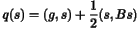subject to the constraint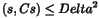where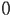denotes the inner product,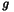is a given vector,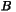is a symmetric linear operator, and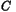is a symmetric and positive definite linear operator
• HCL_UMin_d HCL_UMin_d is the abstract base class for unconstrained minimizers
• HCL_UMin_lbfgs_d HCL_UMin_lbfgs_d implements the limited memory BFGS algorithm for unconstrained minimization
• HCL_UMinAlternation_d HCL_UMinAlternation_d is the simplest concrete class derived from HCL_UMinProductDomain_d
• HCL_UMinNLCG_d HCL_UMinNLCG_d implements the nonlinear conjugate gradient algorithm (Polak-Ribiere form) for unconstrained minimization
• HCL_UMinProductDomain_d HCL_UMinProductDomain_d is the abstract base class for unconstrained minimization algorithms designed for problems in which the objective function is defined on a product space
• HCL_UMinSubspace_d HCL_UMinSubspace_d is a variation on the idea of Kennett for minimizing a function with two or more distinct classes of problem variables
• HCL_UMinTR_d The class HCL_UMinTR_d implements a generic algorithm for solving unconstrained minimization problems using a trust region method
• HCL_UpperTriangularMatOp_d HCL_UpperTriangularMatOp_d makes an upper triangular matrix into a linear operator in the HCL sense
• HCL_Vector_d HCL_Vector_d is the base class for all vectors in HCL
• HCL_VectorSpace_d HCL_VectorSpace_d is the base class for all vector spaces in HCL
• HCL_ZeroBiLinearOp_d HCL_ZeroBiLinearOp_d implements a bilinear operator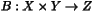which is identically zero: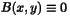• HCL_ZeroLinearOp_d HCL_ZeroLinearOp_d is a class implementing the zero linear operator mapping one vector space to another
• iArray Index arrays for indirect addressing into product spaces and vectors
• SGFSpace_d

SGFSpace objects define the geometry and attributes of uniform rectilinear grids

• SGFVector_d

SGFVector implements sampled functions on a uniform rectilinear grid as HCL_Vectors

• Table ASCII Parameter Table Object

## Functions, Macros

• CheckStability CheckStability should check the appropriate stability condition for the given input vector, returning 1 if the condition is met (that is, if the integration can be done safely) and 0 if the condition fails
• DerivImage DerivImage computes the image of the derivative on dx, yielding dy
• Domain Domain space access
• Domain Domain space access
• Domain Domain returns a reference to the domain of the underlying operator
• Domain Domain space access
• EvalStencilOp_d Usual constructor; requires a pointer to an operator and a reference to the input vector
• Evaluate Evaluate creates an evaluation object which knows how to compute the image and the derivative (as a linear operator with adjoint) at a point
• HCL_delete To be called in place of the operator delete (for HCL objects only)
• Image Image (with no arguments) computes the action of the operator on the vector (c,u) and overwrites u with the result
• Image Image computes the action of the operator on x, giving y
• Image Image computes the image of the operator.
• Image Image compute the action of the operator on x, giving y.
• InitializeControl InitializeControl copies a pointer to the control variable
• InitializeControlPerturbation InitializeControlPerturbation copies a pointer to the control variable
• InvImage InvImage computes the action of the inverse on y, giving x.
• PartialDeriv PartialDeriv computes the partial derivative, with respect to the ith variable, as a linear operator
• PartialDerivAdjImageState PartialDerivAdjImageState computes the image of the adjoint of the partial derivative with respect to the state variable
• PartialDerivImage PartialDerivImage computes the image of the derivative on dx, yielding dy
• PartialDerivImage DerivImage computes the image of the derivative on dx, yielding dy
• PartialDerivImageAddControl PartialDerivImageAddControl computes the image of the derivative, with respect to the control variable, acting on the control perturbation dc
• PartialDerivImageState PartialDerivImageState computes the image of the derivative, with respect to the state variable, acting on the (internal) state perturbation du
• PartialInvImage PartialInvImage computes the image of the inverse, with respect to the second component, of the operator
• Range Range space access
• Range Range returns a reference to the range of the underlying operator.
• Range Range space access
• Range Range space access
• SetTime SetTime sets the time level parameter, which is used in finite difference simulation
• StatePerturbation StatePerturbation returns a reference to the internally-stored state perturbation du; this is a vector in the second factor of the domain
• StateVector StateVector returns a reference to the internally-stored state vector; this is a vector in the second factor of the domain
• Write Debugging information
• Write Debugging information
• Write Debugging information
• Write Debugging information
hierarchy of classes